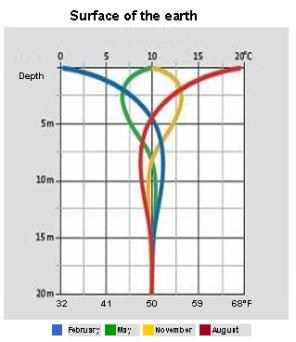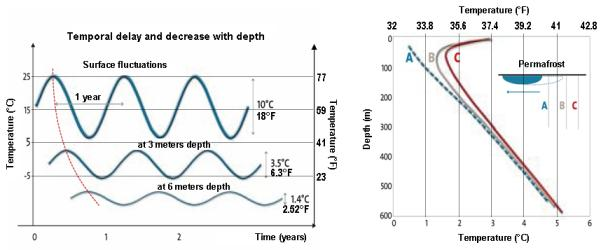Chargement...

# 3) Is human activity powerful enough to explain global warming? 3) Is human activity powerful enough to explain global warming?

3) Is human activity powerful enough to explain global warming?

Between 1975 and 2005, an increase in temperature at the surface level of the earth of at least 0.5 [K] was recorded. Has human activity alone had enough power to generate the heat necessary to warm up the billions of tons of rocks, water and air?

A rigorous calculation would be extremely complex, because in order to compute the quantity of energy corresponding to an increase of 0.5 [K] at the land and ocean surface, we would have to:

• Account for the concomitant increase in energy losses towards space
• Account for the energetic storage that is due to the increase of the water vapor and convection in the atmosphere and the oceans
• Account for the melting of the ice sheets, for the evolution of the cloud cover, and for the modification of the corresponding emissivity of the planet
• Account for the eventual increase in wild forest fires
• Know the vertical change of the temperature within the atmosphere and the thin layer of earth’s crust and oceans concernedThe first figure shows seasonal variation of surface temperatures, which are sensitive mostly down to 15 meters of depth (source: Dimplex).
The second figure (on the left) shows the decrease in temperature fluctuations of the atmosphere with depth and time delay of transmission.
The third figure (on the right) shows:

• In A, the change of temperature nearby a permafrost (underground layer permanently frozen).
• In B and C, the change in temperature in a thawed soil nearby a permafrost, which shows the temperature rise on the first tens of meters of depth.

Source: Paleo-thermal marks of the underground of the BRGM site
For the oceans, the situation fluctuates more, but stratification plays a role as well.

As a first approach, we will carry out a crude estimation by assuming that the increase of 0.5 [K] nearby the ground surface is reflected on all the atmosphere and only half of it is reflected on the first 15 meters of rocks and water below the surface.
In addition, we will not account for the increase in thermal losses towards space, which is suspected to be affected by the increase green house effect.

## Question

Compute in Joule the quantity of heat corresponding to a homogeneous increase of 0.5 [K] on the entire atmosphere and of 0.25 [K] on the first 15 meters of rocks and water below the surface.
Without accounting for the concomitant increase of energy losses towards space, compute the thermal power that corresponds to this warm up recorded over the last 30 years.
Data:

• Mass of the atmosphere:  5.13 x E18 [kg]
• Specific heat capacity of air: 1,000 [J/kg.K]
• Surface of the earth :  5.10 x E14 [m²]
• Average density at the surface of the oceans and of the crust: 1,580 [kg/m³]
• Average specific heat capacity at the surface of the oceans and of the crust: 3,315 [J/kg.K]

Q = 1.26 x E22 [J]
P = 1.33 x E13 [W], which we can compare to the current worldwide power of energy of 1.5 x E13 [W] in 2008.

Explanation:

• Heat corresponding to the warming of 0.5 [K] of the atmosphere

Q = M x C x ΔT  = 5.13 x E18  x 1,000  x  0.5 =  2.57 x E21 [J]

• Heat corresponding to the warming of 0.25 [K] of the first 15 meters from the surface of the oceans and the terrestrial crust:

M = 5.1 x E14 x 15 x 1,580 =  1.21 x E19[kg]
Q = M x C x ΔT  = 1.21 x E19  x 3,315  x  0.25 =  1 x E22 [J]

• Total

Q = 1 x E22 + 2.57 x E21 = 1.26 x E22 [J]

• Power corresponding to a warming in 30 years:

P = Q/t
P = 1.26 x E22 / 365 x 24 x 3,600 x 30 = 1.33 x E13 [W], which we can compare to the current worldwide power of energy of 1.5 x E13 [W] in 2008

Note: accounting for the increase in the quantity of water vapor stocked in the atmosphere during the period modifies the result only very slightly.

Evidently, this computation is too crude to be perfectly correct. It shows however that such a “low” power, such as the one we emit, can explain under specific conditions the current warming “at the surface” of the earth.

But we still need to shed light on a large ambiguity, that is: at the beginning of the last century the planet was subject to an increase in absorbed solar power tens of times superior to the one generated by human activity in 2008 and the resulting increase in temperature was not any greater than a few thenths of degrees.